Download Presentation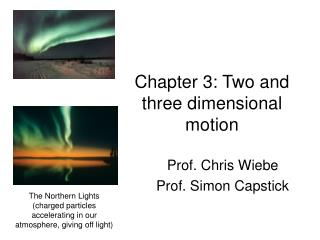Chapter 3: Two and three dimensional motion

# Chapter 3: Two and three dimensional motion - PowerPoint PPT Presentation

Chapter 3: Two and three dimensional motion. Prof. Chris Wiebe Prof. Simon Capstick. The Northern Lights (charged particles accelerating in our atmosphere, giving off light). Chapter Three: 2D and 3D motion.I am the owner, or an agent authorized to act on behalf of the owner, of the copyrighted work described.
Download Presentation## Chapter 3: Two and three dimensional motion

An Image/Link below is provided (as is) to download presentation

Download Policy: Content on the Website is provided to you AS IS for your information and personal use and may not be sold / licensed / shared on other websites without getting consent from its author.While downloading, if for some reason you are not able to download a presentation, the publisher may have deleted the file from their server.

- - - - - - - - - - - - - - - - - - - - - - - - - - E N D - - - - - - - - - - - - - - - - - - - - - - - - - -
Presentation Transcript### Chapter 3: Two and three dimensional motion

Prof. Chris Wiebe

Prof. Simon Capstick

The Northern Lights

(charged particles accelerating in our atmosphere, giving off light)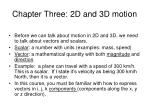Chapter Three: 2D and 3D motion
• Before we can talk about motion in 2D and 3D, we need to talk about vectors and scalars.
• Scalar: a number with units (examples: mass, speed)
• Vector: a mathematical quantity with both magnitude and direction.
• Example: a plane can travel with a speed of 300 km/h. This is a scalar. If I state it’s velocity as being 300 km/h North, then it is a vector.
• In this course, you must be familiar with how to express vectors in i, j, k components (components along the x, y or z direction).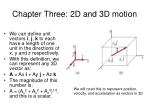Chapter Three: 2D and 3D motion
• We can define unit vectors i, j, k to each have a length of one unit in the directions of x, y and z respectively.
• With this definition, we can represent any 3D vector as:
• A = Ax i + Ay j + Az k
• The magnitude of this number is:
• A = (Ax2 + Ay2 + Az2)1/2, and this is a scalar.

We will need this to represent position, velocity, and acceleration as vectors in 3D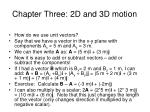Chapter Three: 2D and 3D motion
• How do we use unit vectors?
• Say that we have a vector in the x-y plane with components Ax = 5 m and Ay = 3 m.
• We can then write A as: A = (5 m)i + (3 m)j
• Now it is easy to add or subtract vectors – add or subtract the components!
• If I had a vector B which is Bx= 2 m and By = 1 m, I can add: A + B = (Ax +Bx)i + (Ay + By)j = (5 m + 2 m)i + (3 m + 1 m)j = (7 m)i + (4 m)j
• Exercise: Calculate B – A = (-3 m)i + (-2m)j
• I can also multiply by a scalar: 2A = (2*5 m)i + (2* 3 m)j = (10 m)i + (6 m)j. Note that this just changes the length of the vector (only changes direction if multiplied by a negative scalar).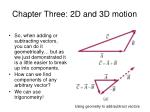Chapter Three: 2D and 3D motion
• So, when adding or subtracting vectors, you can do it geometrically… but as we just demonstrated it is a little easier to break up into components.
• How can we find components of any arbitrary vector?
• We can use trigonometry!

Using geometry to add/subtract vectors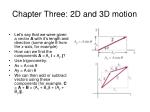Chapter Three: 2D and 3D motion
• Let’s say that we were given a vector A with it’s length and direction (some angle θ from the x-axis, for example)
• How can we find the components A = Axi + Ayj?
• Use trigonometry:
• Ax = A cos θ
• Ay = A sin θ
• We can then add or subtract vectors using these components (for example, C = A + B = (Ax + Bx)i + (Ay + By)j).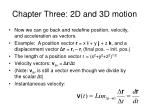Chapter Three: 2D and 3D motion
• Now we can go back and redefine position, velocity, and acceleration as vectors.
• Example: A position vector r = x i + y j + z k, and a displacement vector Δr = rf – ri (final pos. – init. pos.)
• The length of a position vector r = (x2+y2+z2)1/2
• Velocity vectors: vav = Δr/Δt
• (Note: vav is still a vector even though we divide by the scalar Δt)
• Instantaneous velocity: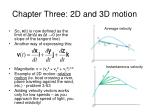Chapter Three: 2D and 3D motion

Average velocity

• So, v(t) is now defined as the limit of Δr/Δt as Δt →0 (or the slope of the tangent line)
• Another way of expressing this:
• Magnitude: v = (vx2 + vy2 + vz2)1/2
• Example of 2D motion: relative motion (ie. boat crossing a river, plane traveling against the wind, etc.) - problem 3-63
• Adding velocity vectors works only for low speeds – as you approach the speed of light, you need relativity!

Instantaneous velocityChapter Three: 2D and 3D motion
• Acceleration!
• Average acceleration vector: aav = Δv/Δt
• Instantaneous acceleration vector:
• Everything becomes a little more confusing with vectors!
• For example: the velocity vector is always pointing in the direction of a particle’s motion.
• The acceleration vector can point in a different direction (it represents the rate ofchange of the velocity vector).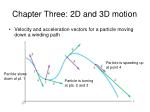Chapter Three: 2D and 3D motion
• Velocity and acceleration vectors for a particle moving down a winding path

Particle is speeding up

at point 4

Particle slows

down at pt. 1

Particle is turning

at pts. 2 and 3